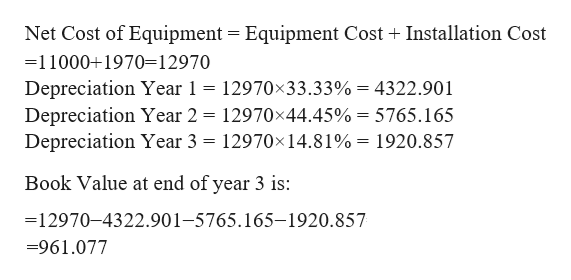Cash flows estimation and capital budgeting: You are the head of finance department in XYZ Company. You are considering adding a new machine to your production facility. The new machine’s  base price is \$11,000.00, and it would cost another \$1,970.00 to install it. The machine falls into the MACRS 3-year class (the applicable MACRS depreciation rates are 33.33%, 44.45%, 14.81%, and 7.41%), and it would be sold after three years for \$1,550.00. The machine would require an increase in net working capital (inventory) of \$840.00. The new machine would not change revenues, but it is expected to save the firm \$41,545.00 per year in before-tax operating costs, mainly labor. XYZ's marginal tax rate is 32.00%.If the project's cost of capital is 17.10%, what is the NPV of the project? Round your answer to two decimal places. For example, if your answer is \$345.667 round as 345.67 and if your answer is .05718 or 5.718% round as 5.72. A. \$41,705.42B. \$52,791.67C. \$11,000.00D. \$12,970.00E. \$30,095.45 a. What is the initial cash outlay? b. What is the free cash flow for year 1? c. What is the additional Year-3 cash flow (i.e, the after-tax salvage and the return of working capital – also called terminal value)?

Question
Cash flows estimation and capital budgeting:
You are the head of finance department in XYZ Company. You are considering adding a new machine to your production facility. The new machine’s  base price is \$11,000.00, and it would cost another \$1,970.00 to install it. The machine falls into the MACRS 3-year class (the applicable MACRS depreciation rates are 33.33%, 44.45%, 14.81%, and 7.41%), and it would be sold after three years for \$1,550.00. The machine would require an increase in net working capital (inventory) of \$840.00. The new machine would not change revenues, but it is expected to save the firm \$41,545.00 per year in before-tax operating costs, mainly labor. XYZ's marginal tax rate is 32.00%.

If the project's cost of capital is 17.10%, what is the NPV of the project?

Round your answer to two decimal places. For example, if your answer is \$345.667 round as 345.67 and if your answer is .05718 or 5.718% round as 5.72.

A. \$41,705.42
B. \$52,791.67
C. \$11,000.00
D. \$12,970.00
E. \$30,095.45

a. What is the initial cash outlay?
b. What is the free cash flow for year 1?
c. What is the additional Year-3 cash flow (i.e, the after-tax salvage and the return of working capital – also called terminal value)?
Step 1

NPV refers to the net present value of an investment. It is calculated as the present value of future cash flows less the initial cash outlay.

Step 2help_outlineImage TranscriptioncloseNet Cost of Equipment Equipment Cost Installation Cost 11000+1970-12970 Depreciation Year 1 12970x33.33% = 4322.901 Depreciation Year 2 12970x44.45% = 5765.165 Depreciation Year 3 = 12970x14.81% = 1920.857 Book Value at end of year 3 is: =12970-4322.901-5765.165-1920.857 -961.077 fullscreen
Step 3

Want to see the full answer?

See Solution

Want to see this answer and more?

Our solutions are written by experts, many with advanced degrees, and available 24/7

See Solution
Tagged in